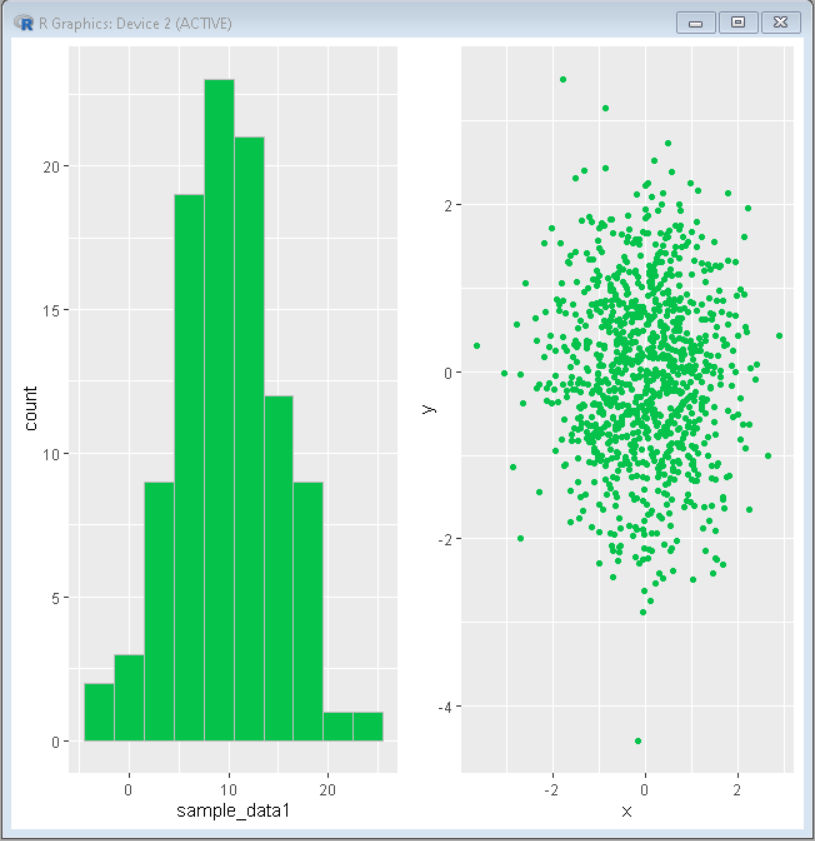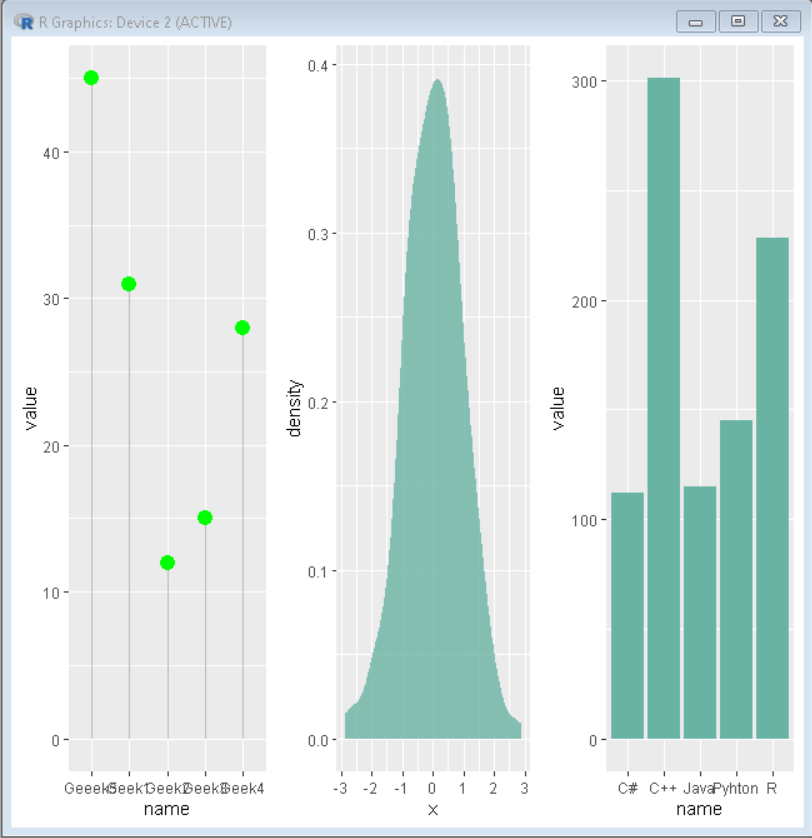Open In App

# Draw Multiple ggplot2 plots Side-by-Side

In the R programming language, we can analyze data by creating different graphs or plot out of them. Sometimes for the analysis, we need to see two or more plots at the same time side by side. In that case, we use gridExtra package of R language that divides the frame into grids, and you can add multiple plots in those grids.

gridExtra contains a function called arrange() that is used to arrange plots as desired.

Syntax:

grid.arrange(plot1, plot2, …, plotn, ncol, nrow)

Parameter:

• plot: some plot
• ncol: number of columns
• nrow: number of rows

To start with this, first create data for all plots. Then, import required packages that will help the requirement. One most important package that needs to installed and imported to the window is gridExtra because without that plots will not be arranged in the desired frame.

Now create and store plots of all the data created. Once the plots are ready, arrange them using arrange() function with the required parameters.

Example 1:

## R

 `# Create sample data``set.seed``(5642)                            ``sample_data1<- ``round``(``rnorm``(100, 10, 5))        ``sample_data2 <- ``data.frame``(x = ``rnorm``(1000),       ``                    ``y = ``rnorm``(1000))` `# Load ggplot2 and gridExtra``library``(``"ggplot2"``)``library``(``"gridExtra"``)` `# Create both plot and store in variable``plot1<-``ggplot``(``data.frame``(sample_data1), ``aes``(sample_data1)) +``geom_histogram``(bins = 10, fill=``"#04c24a"``, color=``"gray"``)` `plot2<- ``ggplot``(sample_data2, ``aes``(x = x, y = y)) + ``geom_point``(color=``"#04c24a"``)` `# Divide frame in grid using grid.arrange function``# and put above created plot int it``grid.arrange``(plot1, plot2, ncol = 2)`

Output:Example 2:

## R

 `# Create sample data``set.seed``(5642)                            ``sample_data1 <- ``data.frame``(name=``c``(``"Geek1"``,``"Geek2"``,``"Geek3"``,``                                  ``"Geek4"``,``"Geeek5"``) ,``                           ``value=``c``(31,12,15,28,45))` `sample_data2 <- ``data.frame``(x = ``rnorm``(400))``sample_data3 <- ``data.frame``(name=``c``(``"C++"``,``"C#"``,``"Java"``,``"R"``,``"Python"``) ,``                           ``value=``c``(301,112,115,228,145))` `# Load ggplot2 and gridExtra``library``(``"ggplot2"``)``library``(``"gridExtra"``)` `# Create both plot and store in variable``plot1<-``ggplot``(sample_data1, ``aes``(x=name, y=value))+``geom_segment``( ``aes``(x=name, xend=name, y=0, yend=value), color=``"grey"``) +``geom_point``( color=``"green"``, size=4)` `plot2<-``ggplot``(sample_data2, ``aes``(x = x)) +``geom_density``(fill=``"#69b3a2"``, color=``"#e9ecef"``, alpha=0.8)` `plot3<-``ggplot``(sample_data3, ``aes``(x=name, y=value)) +``geom_bar``(fill=``"#69b3a2"``, stat = ``"identity"``)` `# Divide frame in grid using grid.arrange function``# and put above created plot int it``grid.arrange``(plot1, plot2,plot3, ncol= 3)`

Output: